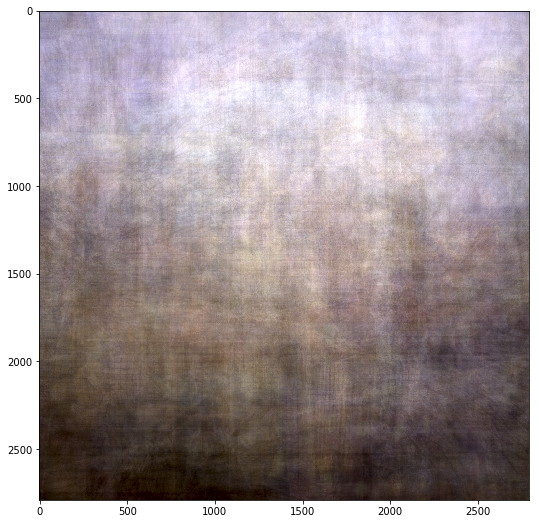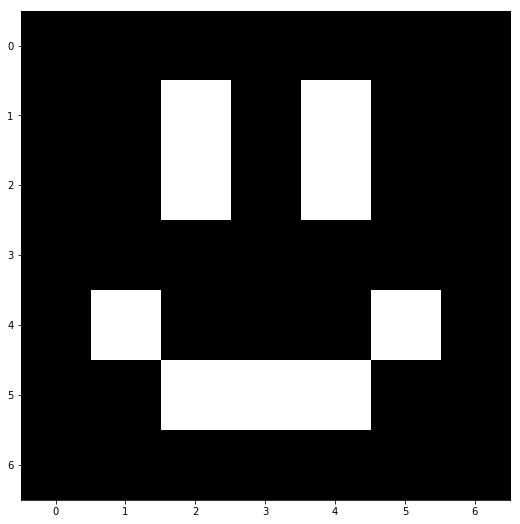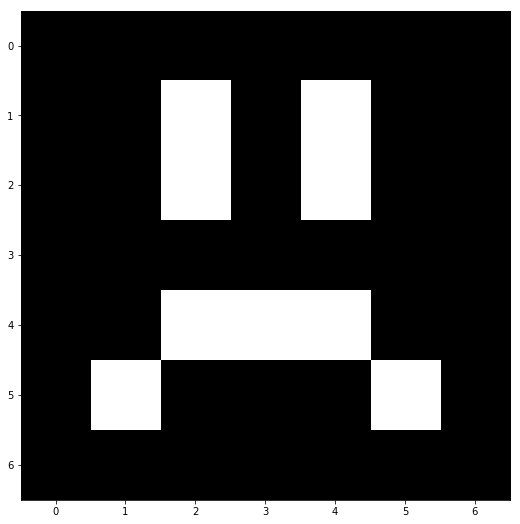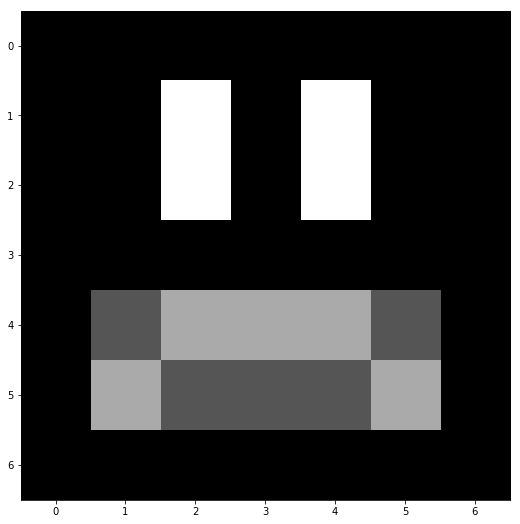# making avg day¶

slides:

### thisisreal.net/talks/20181009_boulder_python/¶

notebook:

andrew catellier

thisisreal.net

for work

• geovisual analytics
• computer vision/machine learning scientist
• us doc—institute for telecommunication science
• "expert"—audio and video quality estimation

for fun:

# what is an average¶

formula for the arithmetic mean:

$$AM={1\over{N}}\sum_{i=1}^{N}a_i \:\text{with}\: a_i \in \mathbb{N}$$

# okay no duh, what's an average image¶

ever seen a double exposure? they're neat.

using python!

## why¶

1. why not
2. because
In :
# pathlib ftw
img_path = Path('/home/jovyan/images/boulder_python/')
avg_img_path = img_path/'avg_march.jpg'

_ = plt.imshow(avg_img)## python image manipulation libraries¶

• PIL (python imaging library)
• quirky api, manipulations done with compiled code
• can read images into a numpy array
• scikit-image
• numpy "native"
• pythonic api
• some manipulations done in python
• openCV (C++ lib with python bindings)
• numpy "native", but can run into undocumented data type (or dtype) issues
• so fast
• a beast to compile

## how to get these libraries¶

pip is good for PIL and scikit-image.

unless you're comfortable compiling libraries, use conda for openCV

if you're not familiar with conda:

• kinda like pip + virtualenv (or pipenv!) on steroids
• probably the best way to install opencv if you really need it
• can use pip to install packages into a conda environment

## start from the very basics: build an array¶

using numpy! and matplotlib!

let's make an image with a smiley face in it.

In :
# make a smile mask

# rows are first index, columns are second
DEMO_IMAGE_SIZE = (7, 7)

Out:
array([[False, False, False, False, False, False, False],
[False, False,  True, False,  True, False, False],
[False, False,  True, False,  True, False, False],
[False, False, False, False, False, False, False],
[False,  True, False, False, False,  True, False],
[False, False,  True,  True,  True, False, False],
[False, False, False, False, False, False, False]])
In :
# make an int image array full of zeros
happy_face = np.zeros(DEMO_IMAGE_SIZE, dtype=np.uint8)

# set anywhere the SMILE_MASK is true to 1 and show it
_ = plt.imshow(happy_face, cmap='binary_r')In :
# make a sad face with twos in itIn :
# now kiss
average_face = (happy_face + sad_face) / 2
_ = plt.imshow(average_face, cmap='binary_r')## now let's try with real images¶

we'll use scikit-image's io module to load the images and then use numpy from there.

In :
# nice pathlib trick
# get all the files with the extension .jpg in the specified folder
input_img_path = img_path/'to_average'
input_image_filename_list = list(input_img_path.glob('*.jpg'))

# load the two files into memory

# show image 0